## Rohan wants to measure the distance of a pond during the visit to his native. He marks points A and B on the opposite edges of a pond as shown in the figure below. To find the distance between the points, he makes a right-angled triangle using rope connecting B with another point C are a distance of 12m, connecting C to point D at a distance of 40m from point C and the connecting D to the point A which is are a distance of 30m from D such the ADC = 900 .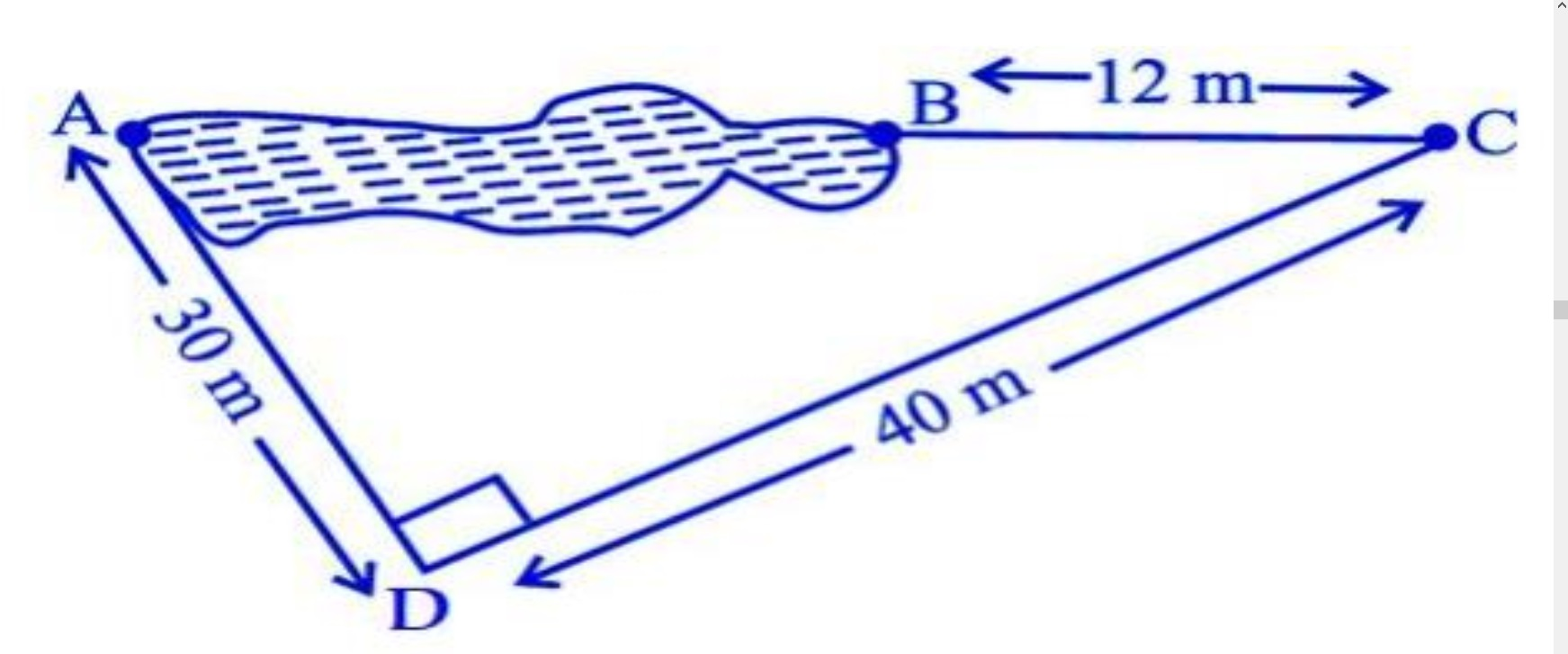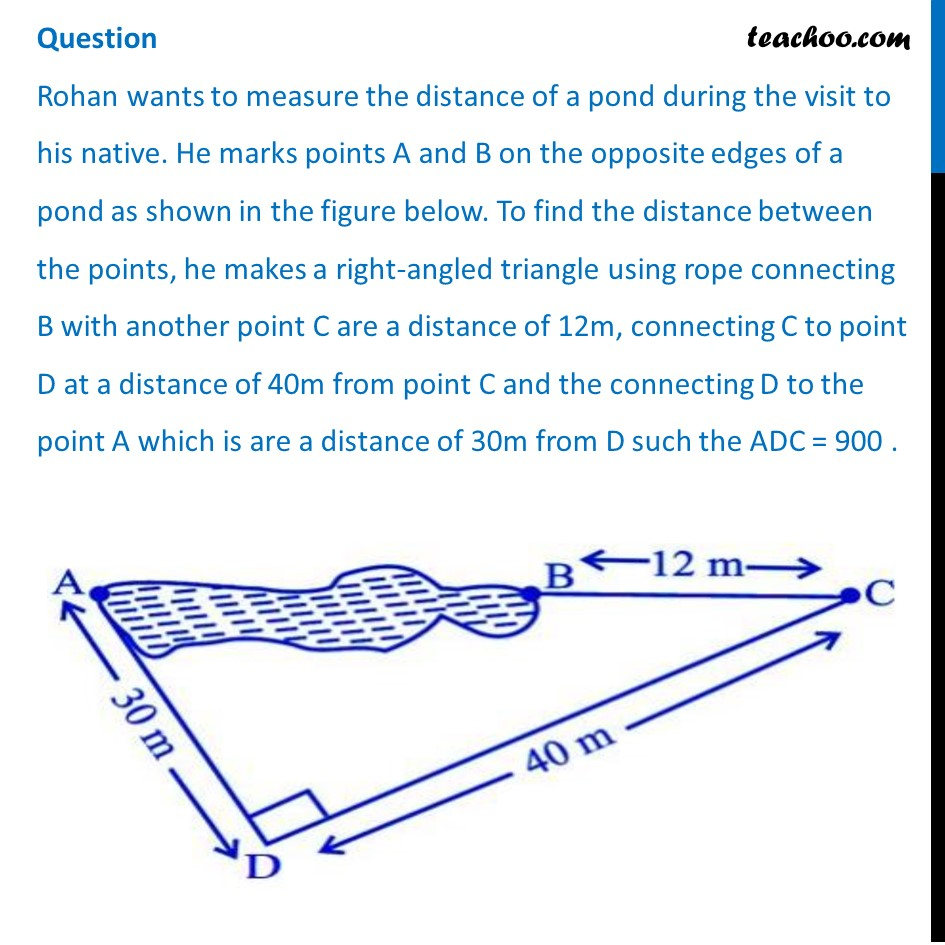## Which property of geometry will be used to find the distance AC? (a) Similarity of triangles (b) Thales Theorem (c) Pythagoras Theorem (d) Area of similar triangles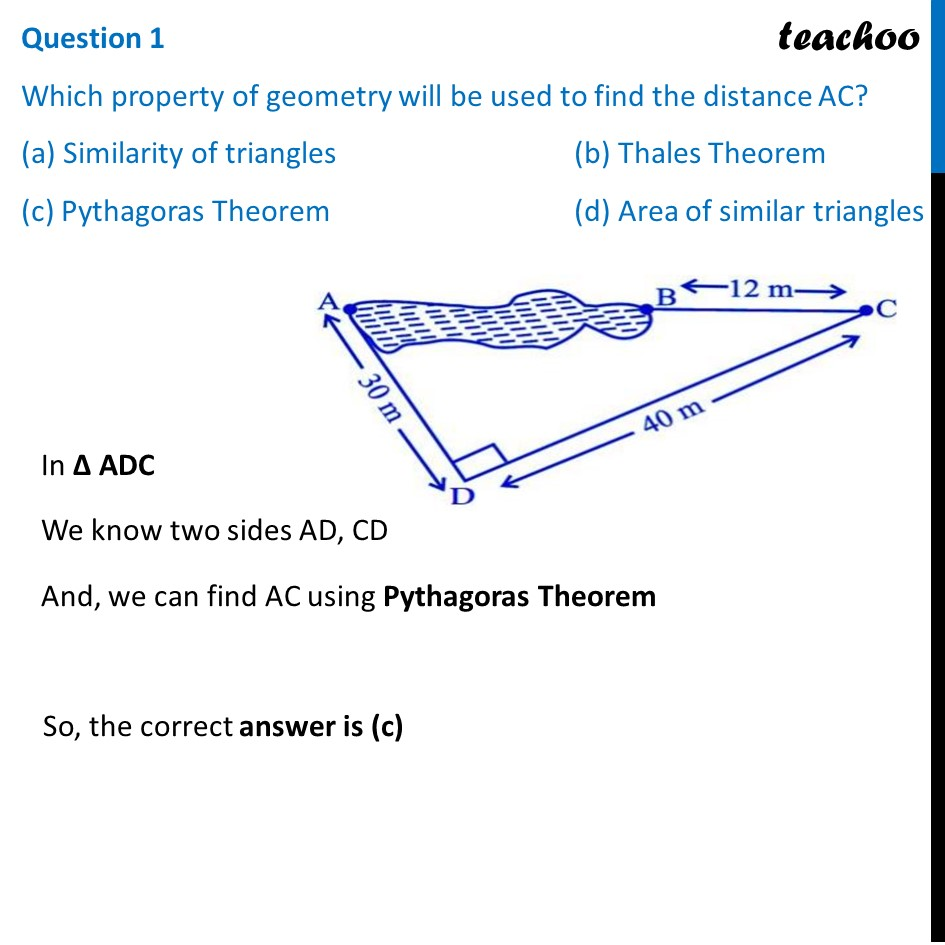## What is the distance AC? (a) 50 m (b) 12 m (c) 100 m (d) 70 m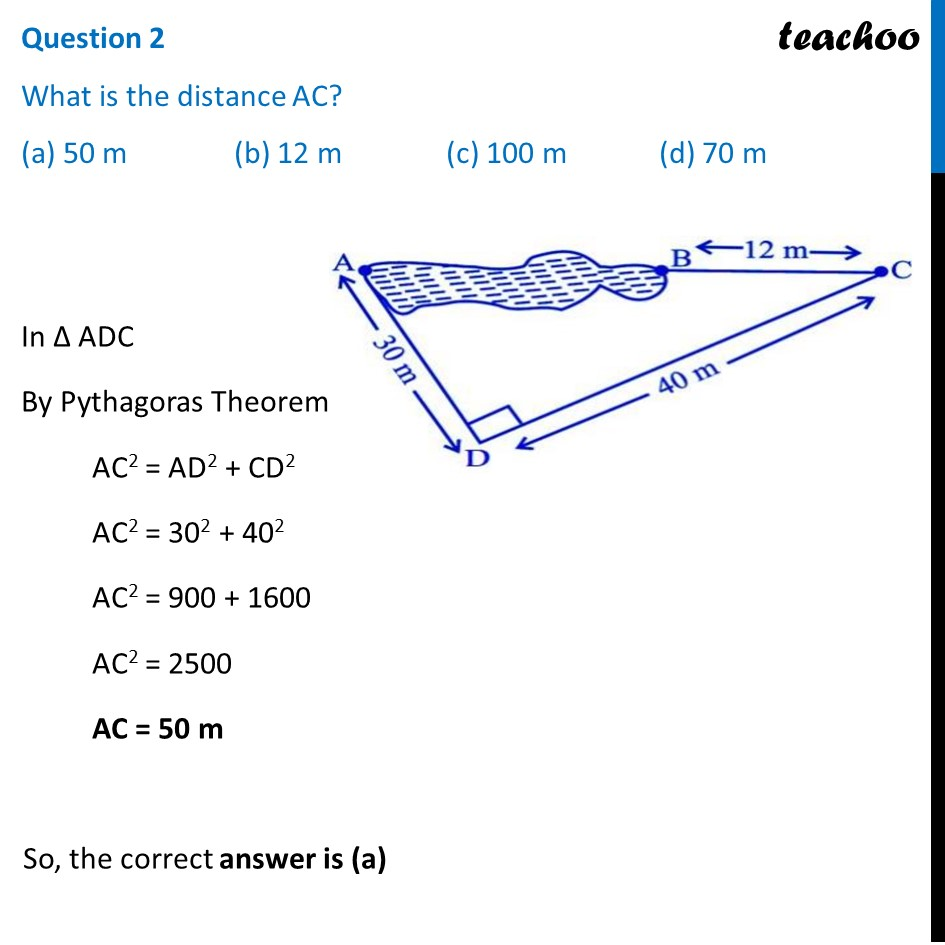## Which is the following does not form a Pythagoras triplet? (a) (7, 24, 25) (b) (15, 8, 17) (c) (5, 12, 13) (d) (21, 20, 28)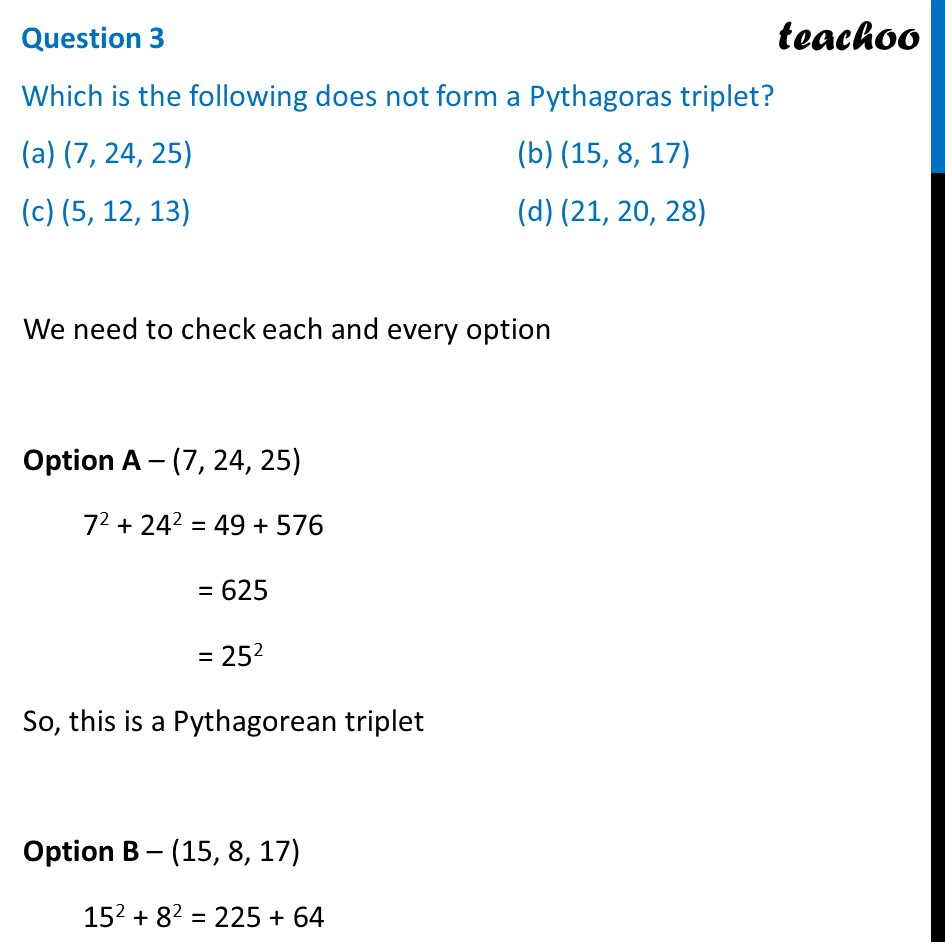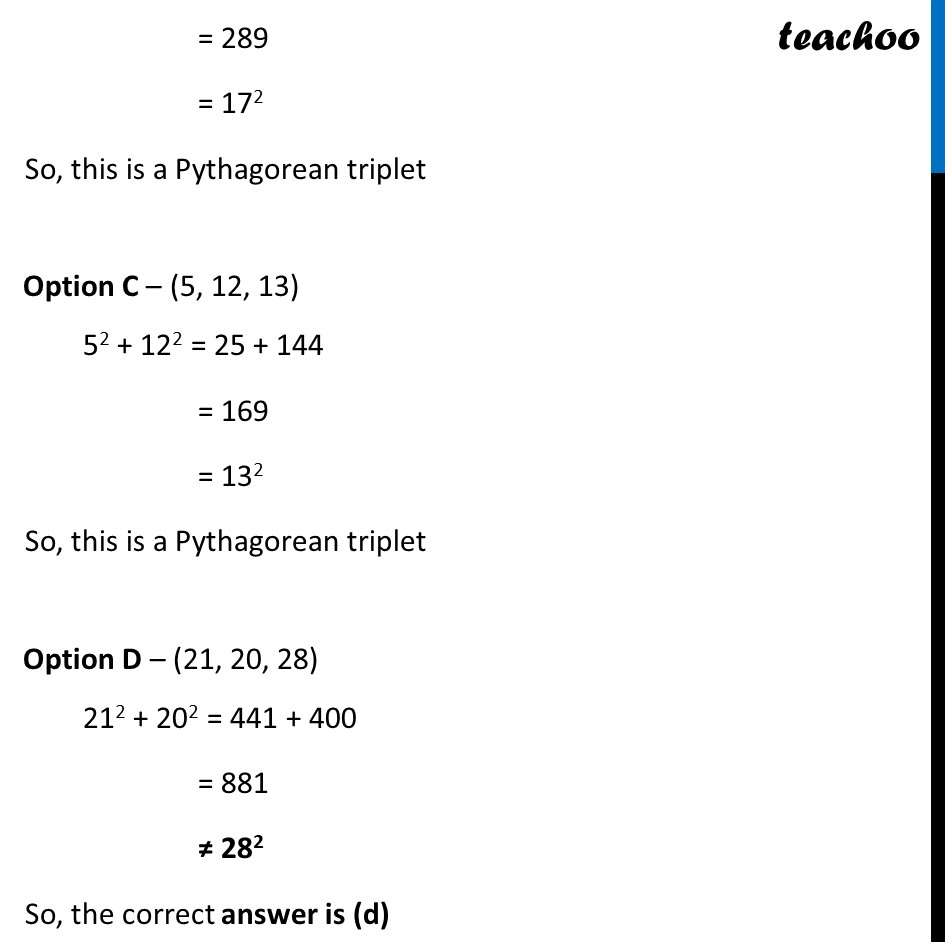## Find the length AB? (a) 12 m (b) 38 m (c) 50 m (d) 100 m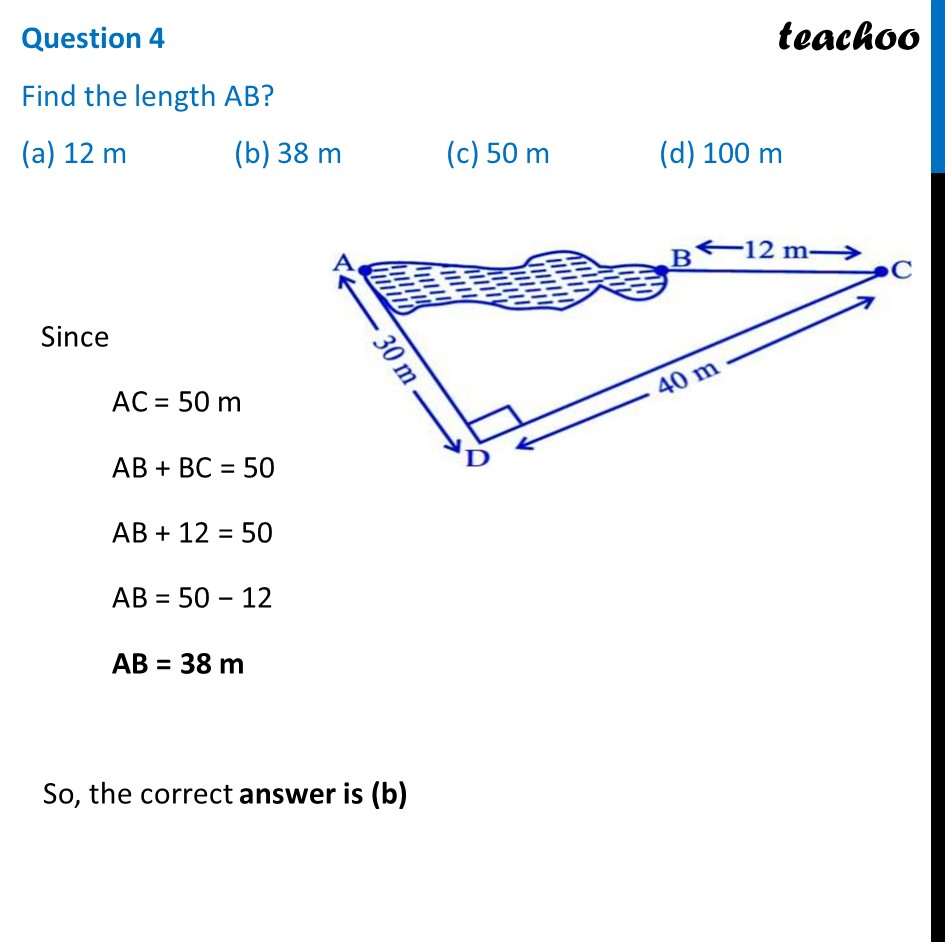## Find the length of the rope used. (a) 120 m (b) 70 m (c) 82 m (d) 22 m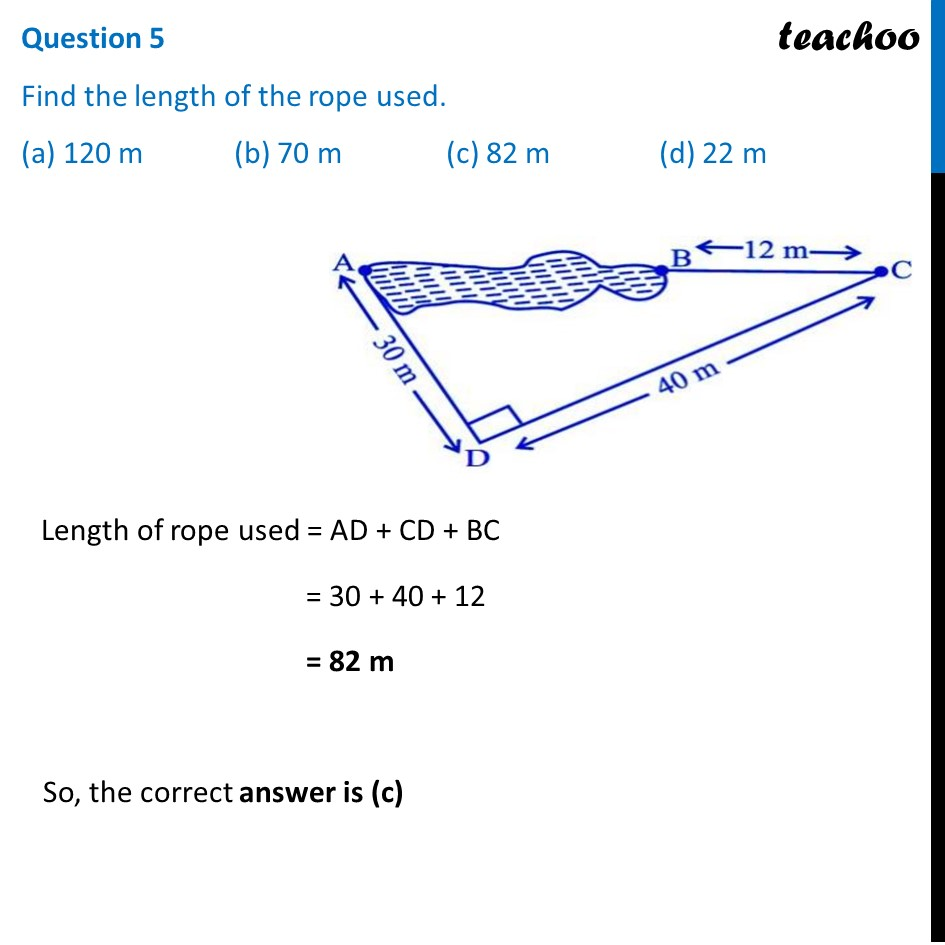1. Chapter 6 Class 10 Triangles (Term 1)
2. Serial order wise
3. Case Based Questions (MCQ)

Transcript

Question Rohan wants to measure the distance of a pond during the visit to his native. He marks points A and B on the opposite edges of a pond as shown in the figure below. To find the distance between the points, he makes a right-angled triangle using rope connecting B with another point C are a distance of 12m, connecting C to point D at a distance of 40m from point C and the connecting D to the point A which is are a distance of 30m from D such the ADC = 900 . Question 1 Which property of geometry will be used to find the distance AC? (a) Similarity of triangles (b) Thales Theorem (c) Pythagoras Theorem (d) Area of similar triangles In Δ ADC We know two sides AD, CD And, we can find AC using Pythagoras Theorem So, the correct answer is (c) Question 2 What is the distance AC? (a) 50 m (b) 12 m (c) 100 m (d) 70 m In Δ ADC By Pythagoras Theorem AC2 = AD2 + CD2 AC2 = 302 + 402 AC2 = 900 + 1600 AC2 = 2500 AC = 50 m So, the correct answer is (a) Question 3 Which is the following does not form a Pythagoras triplet? (a) (7, 24, 25) (b) (15, 8, 17) (c) (5, 12, 13) (d) (21, 20, 28) We need to check each and every option Option A – (7, 24, 25) 72 + 242 = 49 + 576 = 625 = 252 So, this is a Pythagorean triplet Option B – (15, 8, 17) 152 + 82 = 225 + 64 = 289 = 172 So, this is a Pythagorean triplet Option C – (5, 12, 13) 52 + 122 = 25 + 144 = 169 = 132 So, this is a Pythagorean triplet Option D – (21, 20, 28) 212 + 202 = 441 + 400 = 881 ≠ 282 So, the correct answer is (d) Question 4 Find the length AB? (a) 12 m (b) 38 m (c) 50 m (d) 100 m Since AC = 50 m AB + BC = 50 AB + 12 = 50 AB = 50 − 12 AB = 38 m So, the correct answer is (b) Question 5 Find the length of the rope used. (a) 120 m (b) 70 m (c) 82 m (d) 22 m Length of rope used = AD + CD + BC = 30 + 40 + 12 = 82 m So, the correct answer is (c)

Case Based Questions (MCQ)